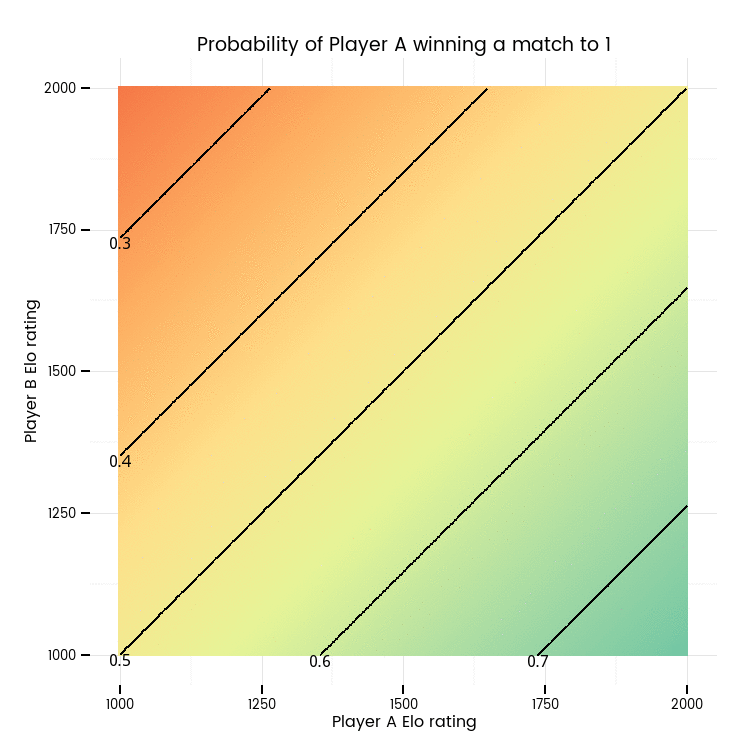# Simulating backgammon players' Elo ratings

## At a glance:

I show how to convert from the Elo ratings of a backgammon player and their opponent and match length to the theoretical probability of winning. I simulate a simple two player backgammon forum to show how Elo ratings vary at random around players 'true' skill level. Along the way, I demonstrate making animated graphics for a webpage.

07 Aug 2015

## Probabilities of winning with a given rating

Backgammon clubs and on-line forums use a modified form of the Elo rating system to keep track of how well individuals have played and draw inferences about their underlying strength. The higher the rating, the stronger the player. Players with higher ratings are inferred to be stronger than those with lower ratings and hence are expected to win; and the longer the match, the more likely the greater skill level will overcome the random chance of the dice. The animated plot below shows the expected probabilities of two players in the FIBS (First Internet Backgammon Server) internet forum, where players start at 1500 and the very best reach over 2000.When the two players have the same rating, they are expected to have a 50/50 chance of winning, and this causes the constant diagonal line in the animation above. When one player is better than the other they have a higher chance of winning, represented for Player A by the increasingly blue space in the bottom right of the plot and the numbers (which are estimated probabilities) labelling the contour lines. The actual formula used on FIBS and illustrated above is defined by on the FIBS site as:

Winning prob. = 1 - (1 / (10 ^ ((YOU - HIM) * SQRT(ML) / 2000) + 1))

(As an aside, I don’t think there is a strong theoretical reason for the SQRT(ML) in that formula. With the complications of the doubling cube I very much doubt that the relationship of the probability winning to match length is as simple as that. But it’s a reasonable empirical approximation; I might blog more about that another time.)

Now, here’s how I made that animation. In the R code below, first, I load up some functionality and the Google font I use for this blog. Then I define a function that estimates the probability of a player winning using the FIBS formula above.

The strategy to create the animation is simple:

• Create all the combinations of two players’ Elo ratings.
• Loop through 23 possible match lengths, calculating the probabilities of A winning for each combination of Elo ratings at that match length
• Draw a still image heatmap for each of those 23 match lengths using ggplot2
• Compile the images into a single animated Gif using ImageMagick.

## Actual ratings varying, “true” rating constant

The estimated probability of winning against a certain opponent at a given match length might be of interest to backgammon players (I’m surprised it’s not referred to more often), but its most common use is embedded in the calculation of how much each player’s Elo rating changes after a match is decided. That formula is well explained by FIBS and a little involved so I won’t spell it out here, but you can see it in action in the function I provide later in this post. A key point for our purposes is that as the win/loss of a match is a random event, players’ Elo ratings which are based on those events are random variables. Even if we grant the existence of a “real” value for each player, it is unobservable, and can only be estimated by their actual Elo rating in a tournament or internet forum.

In fact, my original motivation for this blog post was to see how much Elo ratings fluctuate due to randomness of individual games. In a later post I’ll have a more complex and realistic simulation, but the chart below shows what happens to a player’s Elo ratings over time in the following situation:

• Only two players
• They only ever play 5 point matches, and they play 10,000 of them
• Player A’s chance of winning the 5 point match is 0.6, and this does not change over time (ie no player is improving in skill relative to the other)

The results are shown in the chart below, and in this unrealistically simple scenario there’s more variation than I’d realised there would be. Player A’s actual Elo rating fluctuates fairly wildly around the true value (which can be calculated as 1578.75), hitting 1650 several times and dropping nearly all the way to 1500 at one point.Here’s the code for that simulation, including my R function that provides FIBS-style Elo ratings, adjusted for experience as set out by FIBS.

In this situation, the resulting player’s Elo rating bouncing around at random is quite well modelled by an autoregressive AR(1) time series model with an intercept at the “true” level of the player’s skill. With an autoregression parameter of around 0.98, the rating at any point in time is obviously very closely related to the previous rating; almost but not quite a random walk. Showing how and why would make this post too long, but it’s a useful factoid to note for future work when we come to more realistic and complex simulations of Elo ratings on FIBS.

← Previous post

Next post →